данный урок представляет собой разработку урока алгебры на английском языке. можно применять в полиязычком образовании

### Просмотр содержимого документа«The quadratic inequalities»

M.Kh.Dulati specialized gymnasium #8

with teaching in three languages

Teacher: Pavlenko Yelena Valeryevna

Algebra

Grade 9Aims:

• To develop pupils’ quick wit and logical thinking.

• To make pupils’ work effective through variety of exercises.

• To develop pupils’ skills to practice them on practice.

• To check up the use of different ways of solving the quadratic inequalities.

Aids: interactive blackboard, whiteboard.

Type: ordinary lesson

Plan

I. Org.moment

II. Warm-up. At first let’s answer the questions.

1. Types of inequalities

2. The inequalities are called quadratic if…

3. The basic methods for solving inequalities

4. Find the graphic solution of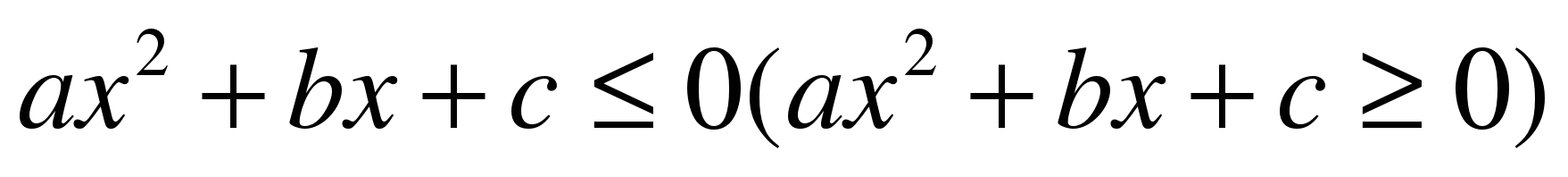1)D0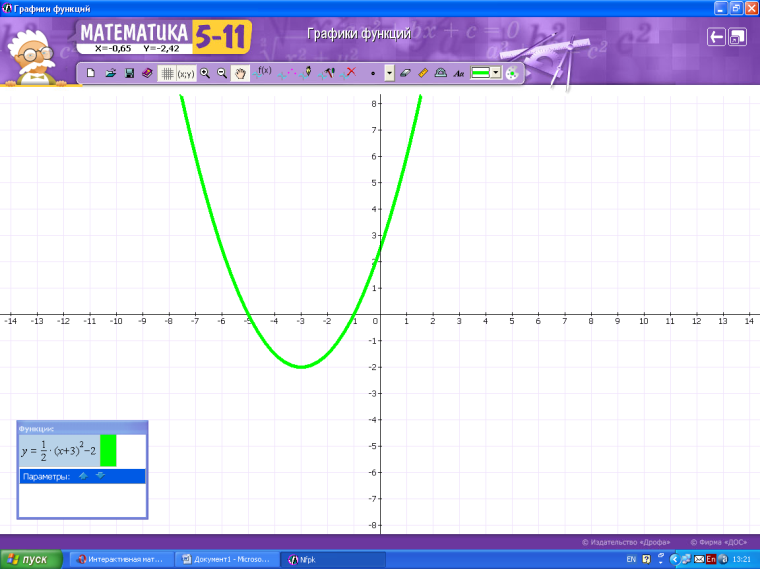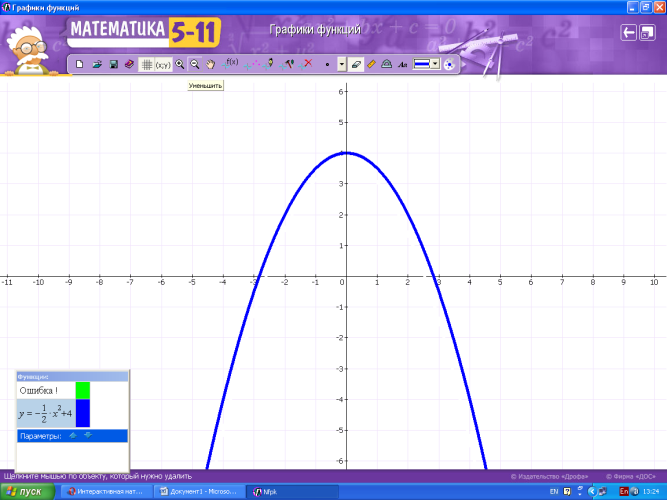2) D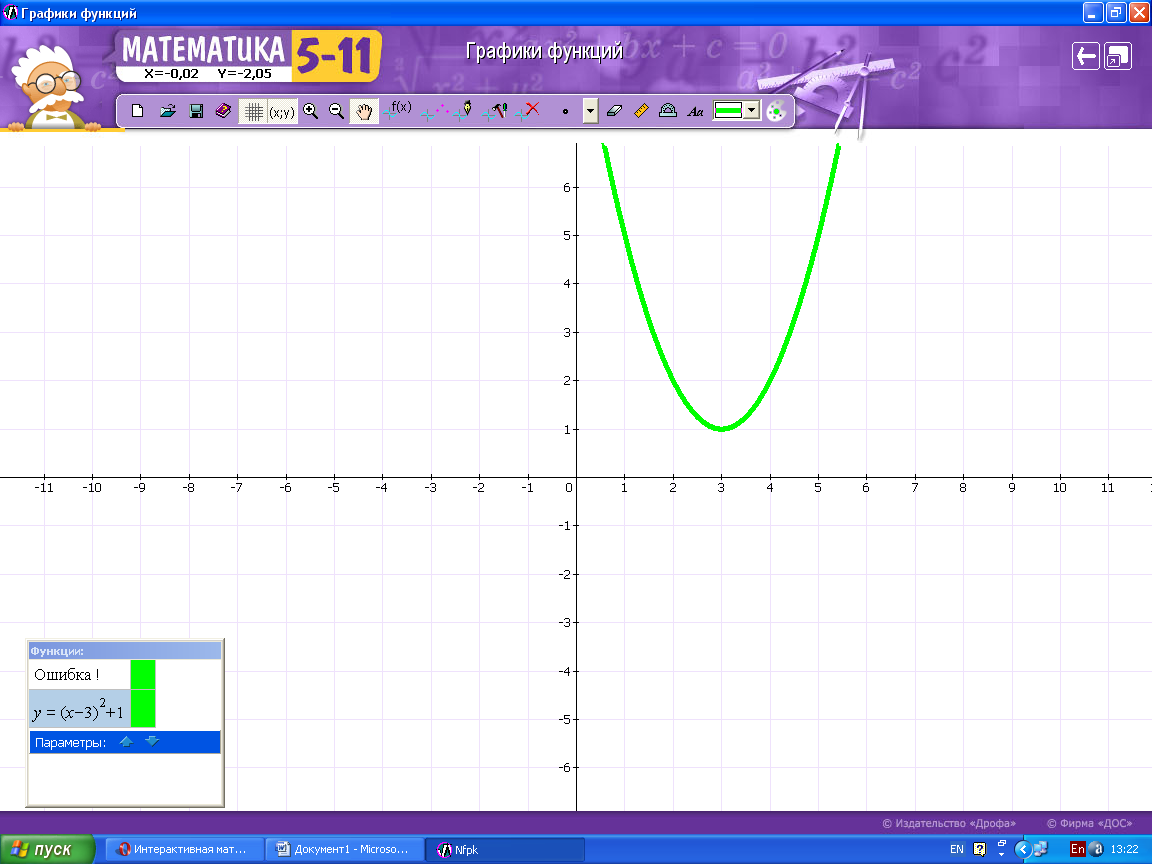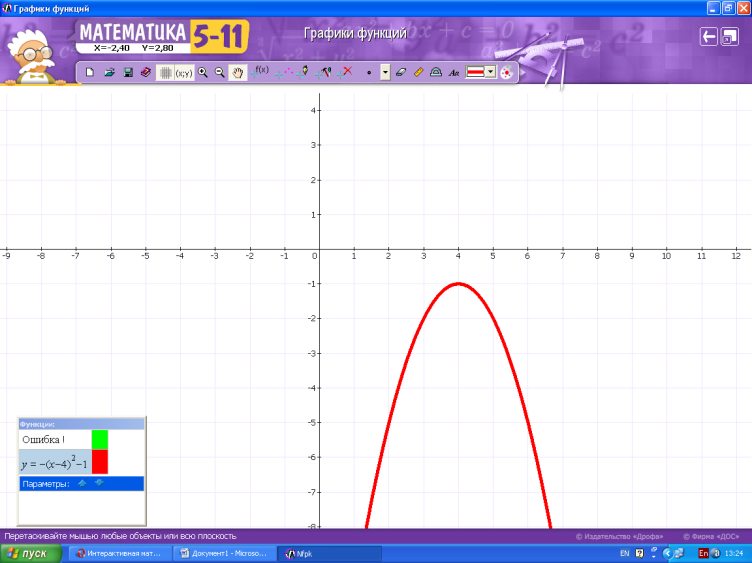3)D=0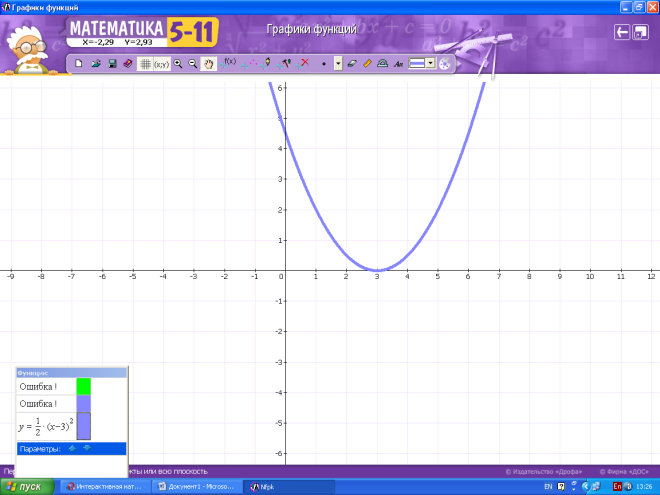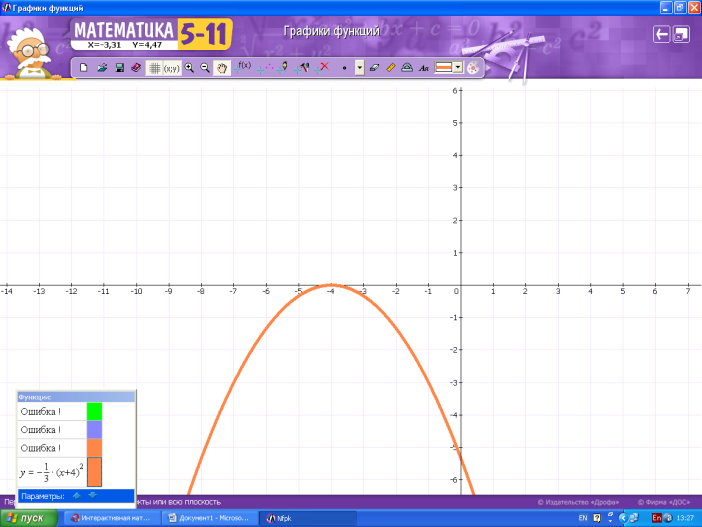1. Which of the graphs shows the solution of the system?

х2 + 5х +6 ≥ у;

х2 + у2 ≤ 16.

1) 2) 3) 4)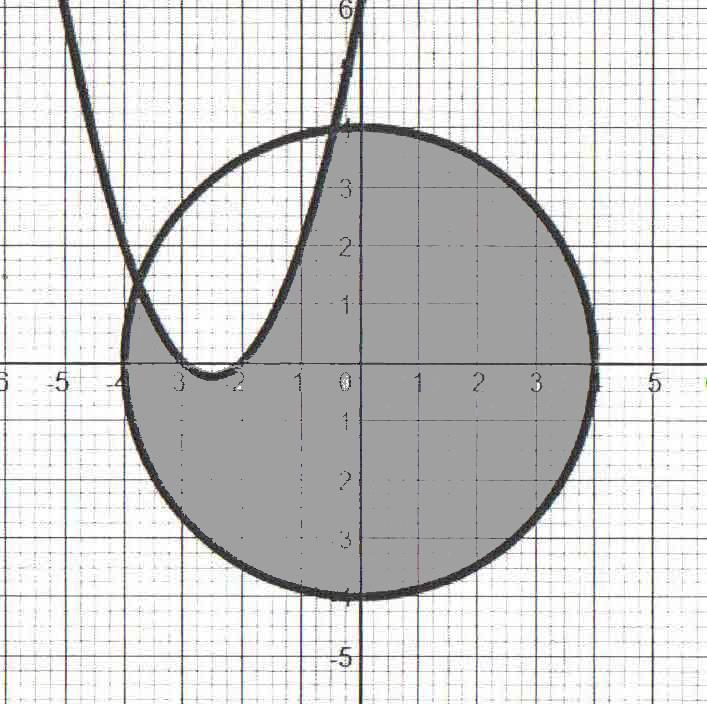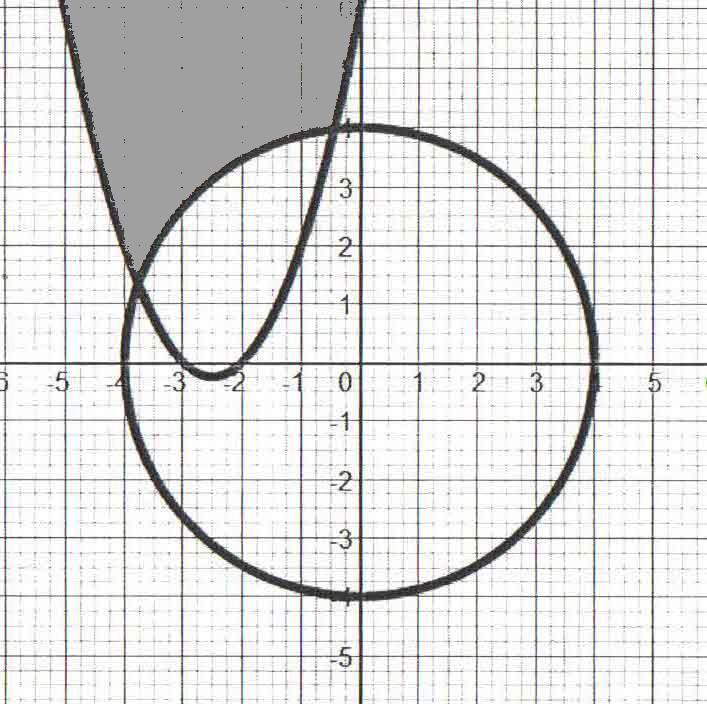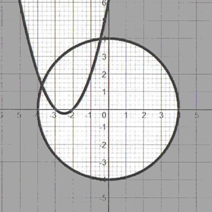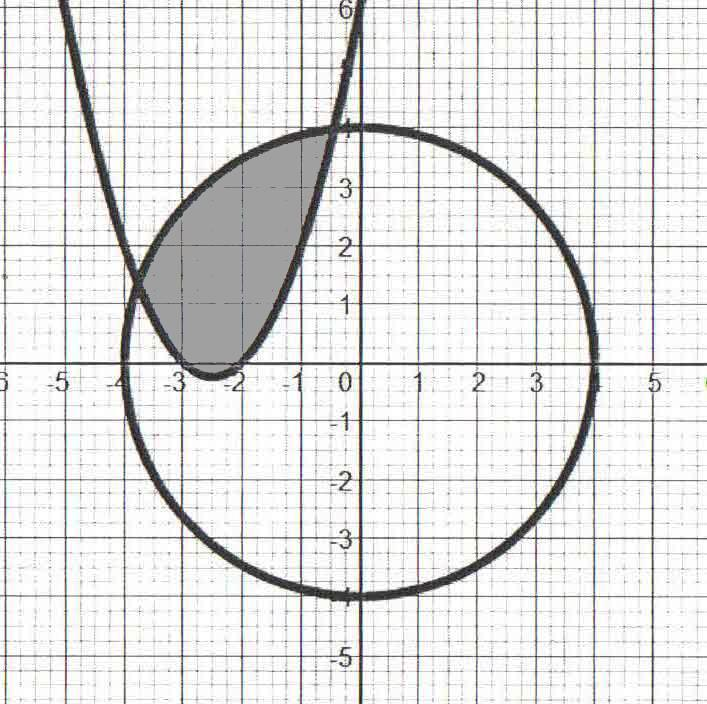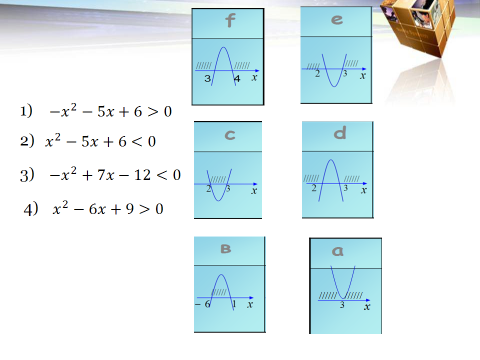III. Solve the problems

1. Solution of inequality. Is it true or false?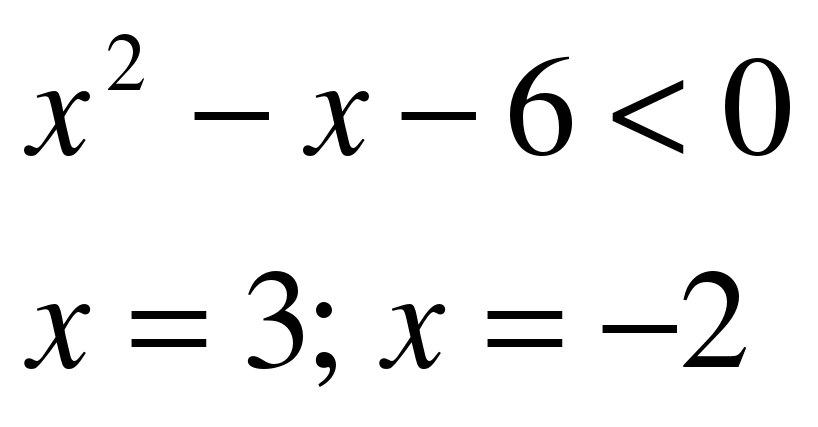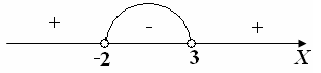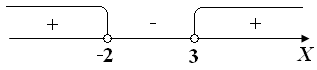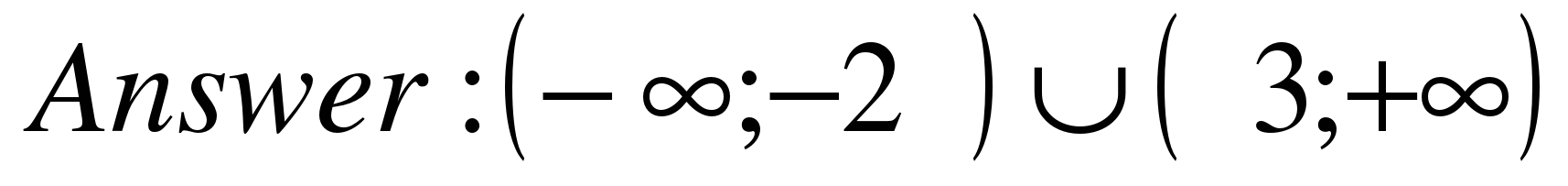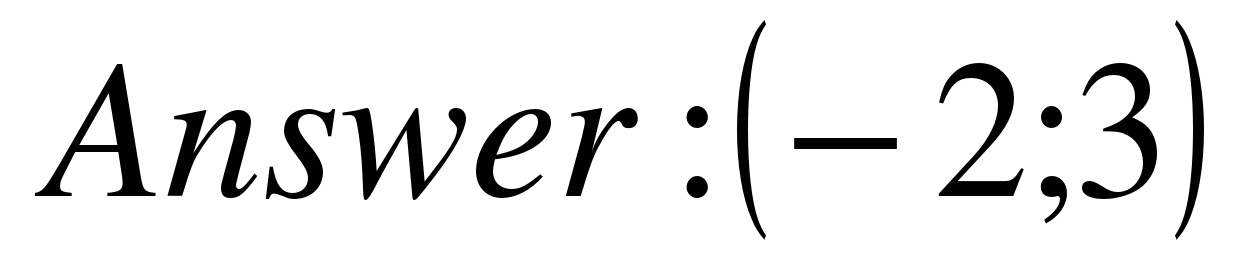1. Solve the problem by using inequalities

1) The area of the right-angled triangle A is greater than the area of the rectangle B. Find the range of possible values of x.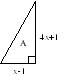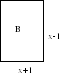Solution: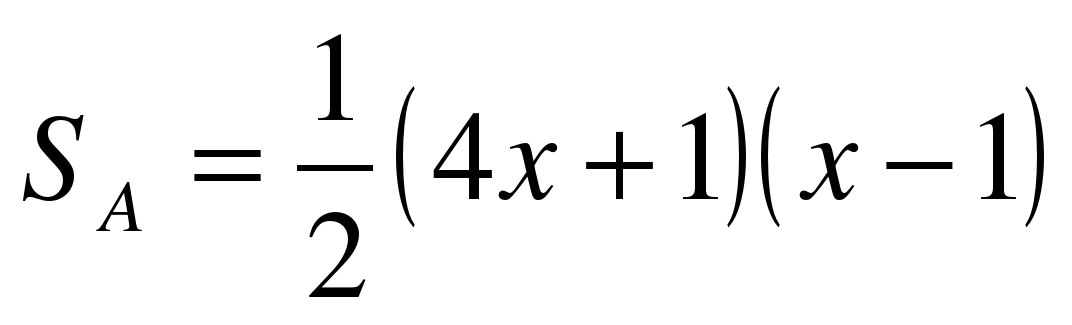The area of the right-angled triangle is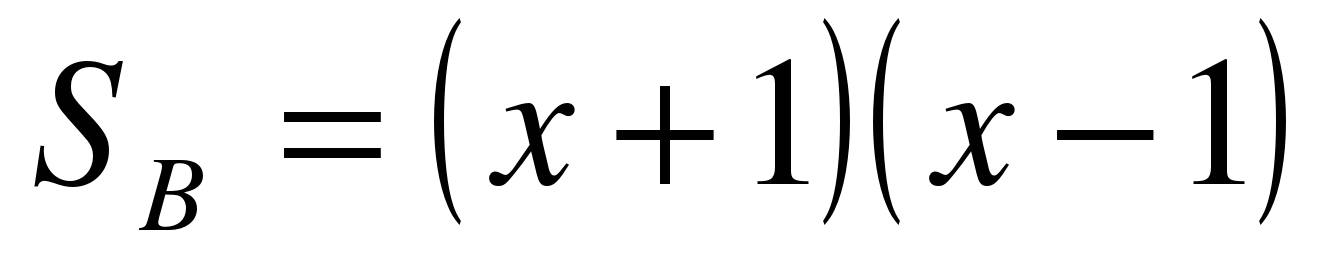The area of the rectangle is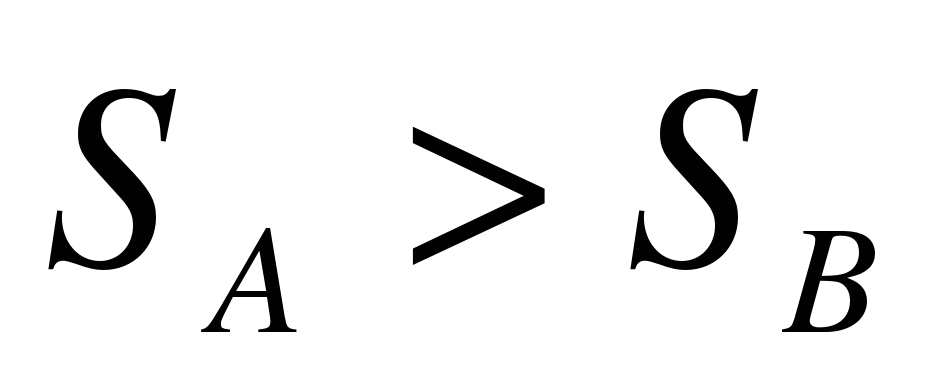As

Plot the solutions on a number line creating the intervals for investigation.  Pick a number from each interval and test it in the original inequality.  If the result is true, that interval is a solution to the inequality.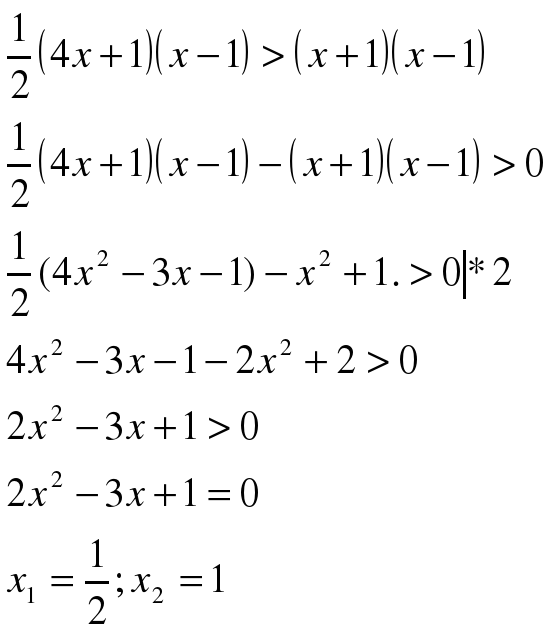The side of triangle and rectangle cannot be equal 0 and negative number, so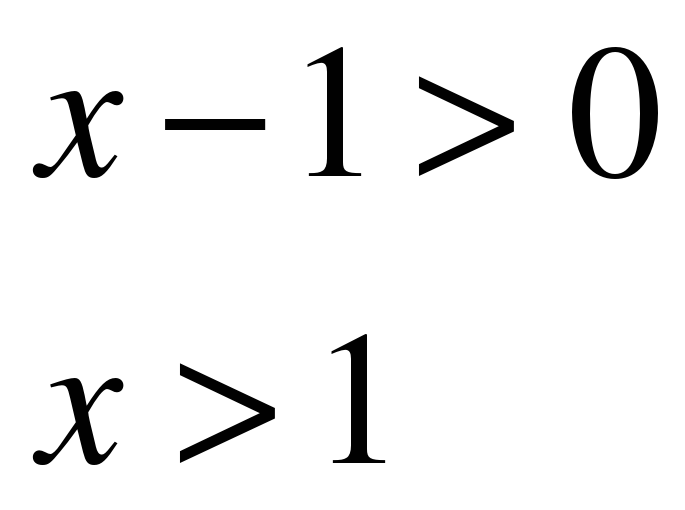Thus the range of possible values of x is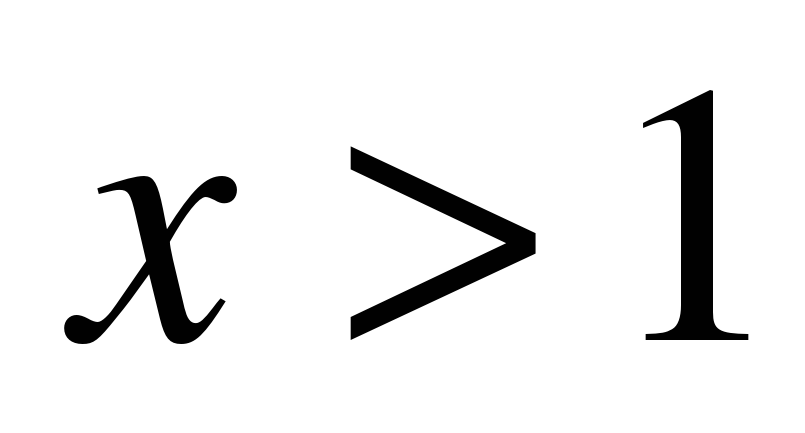Answer:1. A stuntman will jump off a 20 m building. A high-speed camera is ready to film him between 15 m and 10 m above the ground. When should the camera film him?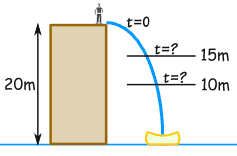We can use this formula for distance and time: d = d0 + v0t + ½a0t2 , where d0=20v0=0, and a0=−9,81, the acceleration due to gravity.

• d = distance above ground (m), and

• t = time from jump (seconds)

d = 20 − 5t2

(Note: if you are curious about the formula, it is simplified from d = d0 + v0t + ½a0t2 ,

OK, let's go.

The distance we want is from 10 m to 15 m:

10

And we know the formula for d:

10 2

Now let's solve it!

First, let's subtract 20 from both sides:

−10 2

Now multiply both sides by −(1/5). But because we are multiplying by a negative number, the inequalities will change direction...

2  t2  1

To be neat, the smaller number should be on the left, and the larger on the right. So let's swap them over (and make sure the inequalities still point correctly):

1 2

Lastly, we can safely take square roots, since all values are greater than zero:

√1

We can tell the film crew: "Film from 1,0 to 1,4 seconds after jumping"

1. Graph the solutions for the following system.

(1) x2 + y2 ≤ 16

(2) y ≤ x2 + 2

Equation (1) is the equation of a circle centered at (0, 0) with a radius of 4. Graph the circle; then select a test point not on the circle and place it into the original inequality. If that result is true, then shade the region where the test point is located. Otherwise, shade the other region. Use (0, 0) as a test point.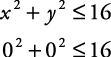This is a true statement. Therefore, the interior of the circle is shaded. In Figure 1(a), this shading is done with horizontal lines.

Equation (2) is the equation of a parabola opening upward with its vertex at (0, 2). Use (0, 0) as a test point.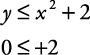This is a true statement. Therefore, shade the exterior of the parabola. In Figure 1(a), this shading is done with vertical lines. The region with both shadings represents the solutions of the systems of inequalities. That solution is shown by the shading on the right side of Figure 1(b).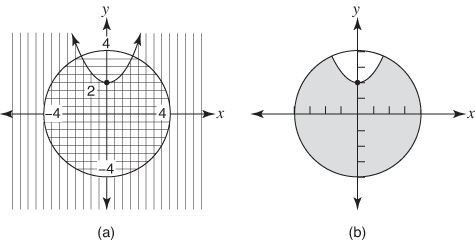Critical thinking questions:

Name one solution to: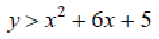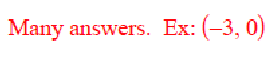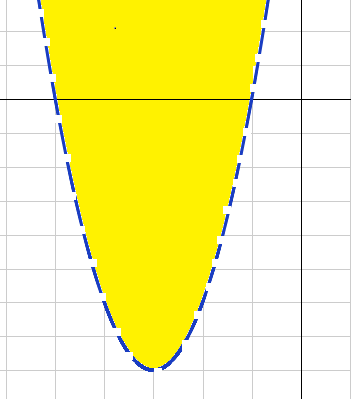IV.

The others you will get another mark at the next lesson.

Thank you for the lesson!

Evaluation sheet. Algebra 9.Grade __________. Date____________

Student_______________________

#

Points

1.

Find the graphic solution of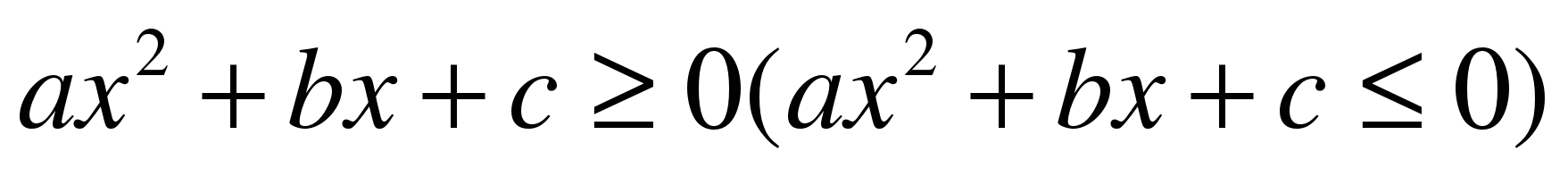2.

 1 2 3 4 5

3.

Find the mistakes in the given solution

4.

Find solutions of the inequality

5.

Solve the problems.

6.

Critical thinking question

Total

Mark

Предмет: Математика

Категория: Уроки

Целевая аудитория: 9 класс.
Урок соответствует ФГОС

Скачать

Автор: Павленко Елена Валерьевна

Дата: 03.12.2016

Номер свидетельства: 365214

ПОЛУЧИТЕ СВИДЕТЕЛЬСТВО МГНОВЕННО

* Свидетельство о публикации выдается БЕСПЛАТНО, СРАЗУ же после добавления Вами Вашей работы на сайт

Удобный поиск материалов для учителей

Ваш личный кабинет
Проверка свидетельства